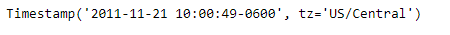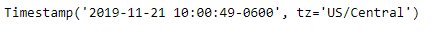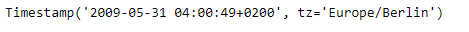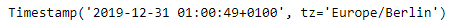# Python | Pandas Timestamp.replace

Python is a great language for doing data analysis, primarily because of the fantastic ecosystem of data-centric python packages. Pandas is one of those packages and makes importing and analyzing data much easier.

Pandas` Timestamp.replace()` function is used to replace the member values of the given Timestamp. The function implements datetime.replace, and it also handles nanoseconds.

Syntax :Timestamp.replace()

Parameters :
year : int
month : int
day : int
hour : int
minute : int
second : int
microsecond : int
nanosecond : int
tzinfo : int
fold : int

Return : Timestamp with fields replaced

Example #1: Use `Timestamp.replace()` function to replace the year value in the given Timestamp.

 `# importing pandas as pd ` `import` `pandas as pd ` ` `  `# Create the Timestamp object ` `ts ``=` `pd.Timestamp(year ``=` `2011``,  month ``=` `11``, day ``=` `21``, ` `                  ``hour ``=` `10``, second ``=` `49``, tz ``=` `'US/Central'``) ` ` `  `# Print the Timestamp object ` `print``(ts) `

Output :Now we will use the `Timestamp.replace()` function to replace the current year in the object with 2019.

 `# replace year ` `ts.replace(year ``=` `2019``) `

Output :As we can see in the output, the `Timestamp.replace()` function has returned a Timestamp object with year value equal to 2019.

Example #2: Use `Timestamp.replace()` function to replace the year, month and hour value in the given Timestamp.

 `# importing pandas as pd ` `import` `pandas as pd ` ` `  `# Create the Timestamp object ` `ts ``=` `pd.Timestamp(year ``=` `2009``, month ``=` `5``, day ``=` `31``, ` `                  ``hour ``=` `4``, second ``=` `49``, tz ``=` `'Europe/Berlin'``) ` ` `  `# Print the Timestamp object ` `print``(ts) `

Output :Now we will use the `Timestamp.replace()` function to replace the current year, month and hour value in the object.

 `# replace year, month and hour value ` `ts.replace(year ``=` `2019``, month ``=` `12``, hour ``=` `1``) `

Output :As we can see in the output, the `Timestamp.replace()` function has returned a Timestamp object with year value equal to 2019, month value equal to 12 and hour value equal to 1.